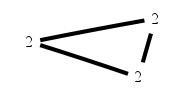# The Markov Bases Database

## uni3-2_bin

Pair interaction model of 3 binary variables.
No threeway interaction model.
Graph model of K3 = C3.

It is a hierarchical model of 3 variables. The dimension of the model is 6.The cardinality of the statespace is 8.

### Properties of the Markov basis

 Markov degree 4 1

 degree 4 1

The model has the following properties:

• All variables are binary.
• It is a graph model.
• The minimal Markov basis is unique.
• The semigroup is normal.### FILES

 Markov basis: sufficient statistics matrix: uni3-2_bin.mar (29 b) uni3-2_bin.mat (209 b) uni3-2_bin.mod (30 b) uni3-2_bin.tar.gz (4.25 kb)

Wrong or missing information? Write us an email.

page last update on November 15 2020. ->Contact